#### 相似文章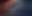# 数据科学和机器学习（第 12 部分）：自训练神经网络能否帮助您跑赢股市？

MetaTrader 5交易 | 7 八月 2023, 15:061 1470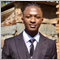“我并不是说神经网络很容易。 您需要成为专家才能令这些事情发挥作用。 但是，这些专业知识可在更广泛的应用中为您提供服务。 从某种意义上说，以前用于特征设计的所有努力现在都投入到架构设计、损失函数设计、以及优化方案设计当中。 手工劳动已经提升到更高的抽象水平。“

### 概述### 前向验算

1. 回归问题
2. 分类问题

01： 回归问题

02： 分类问题

```matrix CRegNeuralNets::ForwardPass(vector &input_v)
{
matrix INPUT = this.matrix_utils.VectorToMatrix(input_v);
matrix OUTPUT;

OUTPUT = W.MatMul(INPUT); //Weight X Inputs

OUTPUT = OUTPUT + B;  //Outputs + Bias

OUTPUT.Activation(OUTPUT, ENUM_ACTIVATION_FUNCTION(A_FX)); //Activation Function

return (OUTPUT);
}```

`matrix INPUT = this.matrix_utils.VectorToMatrix(input_v);`

• 第一个神经网络输入矩阵是 nx1 矩阵
• 权重矩阵为 HN x n；其中 HN 是当前隐藏层中的节点数，n 是来自前一层的输入数或来自输入矩阵的行数。
• 偏置矩阵的大小与层的输出大小相同。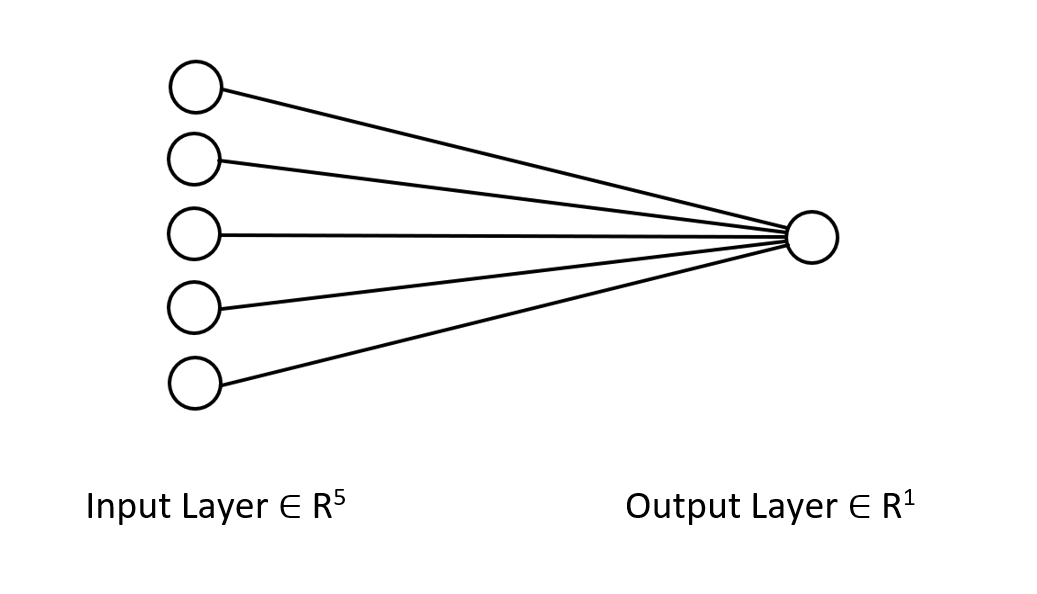### 生成权重

`   this.W = matrix_utils.Random(0.0, 1.0,1,m_inputs, RANDOM_STATE);`

Xavier/Glorot 初始化最适合 sigmoid 和 tanh 激活函数，而 He 初始化适用于 RELU 及其变体。

He 初始化

Xavier 初始化方法计算为具有均匀正态分布（U）的随机数。 其公式如下：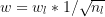```   this.W = matrix_utils.Random(0.0, 1.0,1,m_inputs, RANDOM_STATE);
this.W = this.W * 1/sqrt(m_inputs); //He initialization```

### 激活函数

```enum activation
{
AF_ELU_ = AF_ELU,
AF_EXP_ = AF_EXP,
AF_GELU_ = AF_GELU,
AF_LINEAR_ = AF_LINEAR,
AF_LRELU_ = AF_LRELU,
AF_RELU_ = AF_RELU,
AF_SELU_ = AF_SELU,
AF_TRELU_ = AF_TRELU,
AF_SOFTPLUS_ = AF_SOFTPLUS
};
```

### 损失函数

```enum loss
{
LOSS_MSE_ = LOSS_MSE,
LOSS_MAE_ = LOSS_MAE,
LOSS_MSLE_ = LOSS_MSLE,
LOSS_HUBER_ = LOSS_HUBER
};```

### 使用增量规则的反向传播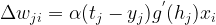是一个称为学习率的小常数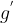是 g 的导数

g(x)  是神经元的激活函数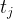是目标输出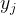是实际输出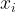是第 i 个输入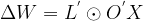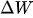= 权重矩阵的变化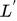= 损失函数的导数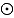=  逐元素矩阵乘法/哈达玛（Hadamard）乘积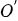= 神经元激活矩阵的导数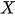= 输入矩阵。

L 矩阵始终与 O 矩阵具有相同的大小，并且右侧生成的矩阵需要与 W 矩阵具有相同的大小。 否则，您就抓瞎了。

```for (ulong iter=0; iter<m_rows; iter++)
{
OUTPUT = ForwardPass(m_x_matrix.Row(iter)); //forward pass
pred = matrix_utils.MatrixToVector(OUTPUT);

actual = m_y_vector[iter];

preds[iter] = pred;
actuals[iter] = actual;

//---

INPUT = matrix_utils.VectorToMatrix(m_x_matrix.Row(iter));

LOSS_DX.Col(loss_v, 0);

OUTPUT.Derivative(OUTPUT, ENUM_ACTIVATION_FUNCTION(A_FX));

OUTPUT = LOSS_DX * OUTPUT;

INPUT = INPUT.Transpose();
DX_W = OUTPUT.MatMul(INPUT);

this.W -= (alpha * DX_W); //Weights update by gradient descent
}```

Epochs：单个 epoch 是指整个数据集完全向前和向后循环贯穿网络。 简单来说，当网络看到所有数据时。 世代数量越大，训练神经网络所需的时间就越长，学习效果就越好。

Alpha： 是您希望梯度下降算法在达到全局和局部最小值时采用的步长大小。 Alpha 通常是介于 0.1 和 0.00001 之间的小值。 此值越大，网络收敛越快，但跳过局部最小值的风险就越高。

```   for (ulong epoch=0; epoch<epochs && !IsStopped(); epoch++)
{
for (ulong iter=0; iter<m_rows; iter++)
{
OUTPUT = ForwardPass(m_x_matrix.Row(iter));
pred = matrix_utils.MatrixToVector(OUTPUT);

actual = m_y_vector[iter];

preds[iter] = pred;
actuals[iter] = actual;

//---

INPUT = matrix_utils.VectorToMatrix(m_x_matrix.Row(iter));

LOSS_DX.Col(loss_v, 0);

OUTPUT.Derivative(OUTPUT, ENUM_ACTIVATION_FUNCTION(A_FX));

OUTPUT = LOSS_DX * OUTPUT;

INPUT = INPUT.Transpose();
DX_W = OUTPUT.MatMul(INPUT);

this.W -= (alpha * DX_W);
}

printf("[ %d/%d ] Loss = %.8f | accuracy %.3f ",epoch+1,epochs,preds.Loss(actuals,ENUM_LOSS_FUNCTION(L_FX)),metrics.r_squared(actuals, preds));
}```

```#include <MALE5\Neural Networks\selftrain NN.mqh>
#include <MALE5\matrix_utils.mqh>

CRegNeuralNets *nn;
CMatrixutils matrix_utils;
//+------------------------------------------------------------------+
//| Expert initialization function                                   |
//+------------------------------------------------------------------+
int OnInit()
{
//---
matrix Matrix = {
{1,2,3},
{2,3,5},
{3,4,7},
{4,5,9},
{5,6,11}
};

matrix x_matrix; vector y_vector;

matrix_utils.XandYSplitMatrices(Matrix,x_matrix,y_vector);

nn = new CRegNeuralNets(x_matrix,y_vector,0.01,100, AF_RELU_, LOSS_MSE_); //Training the Network

//---
return(INIT_SUCCEEDED);
}```

X 矩阵 Y 向量
{ {1, 2},
{2, 3},
{3, 4},
{4, 5},
{5, 6} }
{3},
{5},
{7},
{9},
{11}

```CS      0       20:30:00.878    Self Trained NN EA (Apple_Inc_(AAPL.O),H1)      [1/100] Loss = 56.22401001 | accuracy -6.028
CS      0       20:30:00.879    Self Trained NN EA (Apple_Inc_(AAPL.O),H1)      [2/100] Loss = 2.81560904 | accuracy 0.648
CS      0       20:30:00.879    Self Trained NN EA (Apple_Inc_(AAPL.O),H1)      [3/100] Loss = 0.11757813 | accuracy 0.985
CS      0       20:30:00.879    Self Trained NN EA (Apple_Inc_(AAPL.O),H1)      [4/100] Loss = 0.01186759 | accuracy 0.999
CS      0       20:30:00.879    Self Trained NN EA (Apple_Inc_(AAPL.O),H1)      [5/100] Loss = 0.00127888 | accuracy 1.000
CS      0       20:30:00.879    Self Trained NN EA (Apple_Inc_(AAPL.O),H1)      [6/100] Loss = 0.00197030 | accuracy 1.000
CS      0       20:30:00.879    Self Trained NN EA (Apple_Inc_(AAPL.O),H1)      [7/100] Loss = 0.00173890 | accuracy 1.000
CS      0       20:30:00.879    Self Trained NN EA (Apple_Inc_(AAPL.O),H1)      [8/100] Loss = 0.00178597 | accuracy 1.000
CS      0       20:30:00.879    Self Trained NN EA (Apple_Inc_(AAPL.O),H1)      [9/100] Loss = 0.00177543 | accuracy 1.000
CS      0       20:30:00.879    Self Trained NN EA (Apple_Inc_(AAPL.O),H1)      [10/100] Loss = 0.00177774 | accuracy 1.000
…
…
…
CS      0       20:30:00.883    Self Trained NN EA (Apple_Inc_(AAPL.O),H1)      [100/100] Loss = 0.00177732 | accuracy 1.000 ```

```   vector new_data = {7,8};

Print("Test ");
Print(new_data," pred = ",nn.ForwardPass(new_data));
```

```CS      0       20:37:36.331    Self Trained NN EA (Apple_Inc_(AAPL.O),H1)      Test
CS      0       20:37:36.331    Self Trained NN EA (Apple_Inc_(AAPL.O),H1)      [7,8] pred = [[14.96557787153696]]```

```input string symbol_x = "Apple_Inc_(AAPL.O)";
input string symbol_x2 = "Tesco_(TSCO.L)";

input ENUM_COPY_RATES copy_rates_x = COPY_RATES_OPEN;
input int n_samples = 100;

//+------------------------------------------------------------------+
//| Expert initialization function                                   |
//+------------------------------------------------------------------+
int OnInit()
{

matrix x_matrix(n_samples,2); vector y_vector;

vector x_vector;
//---
x_vector.CopyRates(symbol_x,PERIOD_CURRENT,copy_rates_x,0,n_samples);
x_matrix.Col(x_vector, 0);
x_vector.CopyRates(symbol_x2, PERIOD_CURRENT,copy_rates_x,0,n_samples);
x_matrix.Col(x_vector, 1);

y_vector.CopyRates(Symbol(), PERIOD_CURRENT,COPY_RATES_CLOSE,0,n_samples);

nn = new CRegNeuralNets(x_matrix,y_vector,0.01,1000, AF_RELU_, LOSS_MSE_);
```

```CS      0       21:29:20.698    Self Trained NN EA (NAS100,M30) [ 1/1000 ] Loss = 353809311769.08959961 | accuracy -27061631.733
CS      0       21:29:20.698    Self Trained NN EA (NAS100,M30) [ 2/1000 ] Loss = 149221473.48209998 | accuracy -11412.427
CS      0       21:29:20.699    Self Trained NN EA (NAS100,M30) [ 3/1000 ] Loss = 149221473.48209998 | accuracy -11412.427
CS      0       21:29:20.699    Self Trained NN EA (NAS100,M30) [ 4/1000 ] Loss = 149221473.48209998 | accuracy -11412.427
CS      0       21:29:20.699    Self Trained NN EA (NAS100,M30) [ 5/1000 ] Loss = 149221473.48209998 | accuracy -11412.427
CS      0       21:29:20.699    Self Trained NN EA (NAS100,M30) [ 6/1000 ] Loss = 149221473.48209998 | accuracy -11412.427
....
....
CS      0       21:29:20.886    Self Trained NN EA (NAS100,M30) [ 1000/1000 ] Loss = 149221473.48209998 | accuracy -11412.427
```

### 最小-最大缩放器

x_norm = (x -x_min) / (x_max - x_min)

x = 原始特征

x_min = 要素的最小值

x_max = 要素的最大值

x_norm = 新规范化的要素值

`CRegNeuralNets::CRegNeuralNets(matrix &xmatrix, vector &yvector,double alpha, uint epochs, activation ACTIVATION_FUNCTION, loss LOSS_FUNCTION, norm_technique NORM_METHOD)`

```enum norm_technique
{
NORM_MIN_MAX_SCALER, //Min max scaler
NORM_MEAN_NORM,  //Mean normalization
NORM_STANDARDIZATION, //standardization
NORM_NONE    //Do not normalize.
};
```

`   nn = new CRegNeuralNets(x_matrix,y_vector,0.01,1000, AF_RELU_, LOSS_MSE_,NORM_MIN_MAX_SCALER);`

```CS      0       22:40:56.457    Self Trained NN EA (NAS100,M30) [ 1/1000 ] Loss = 0.19379434 | accuracy -0.581
CS      0       22:40:56.457    Self Trained NN EA (NAS100,M30) [ 2/1000 ] Loss = 0.07735744 | accuracy 0.369
CS      0       22:40:56.458    Self Trained NN EA (NAS100,M30) [ 3/1000 ] Loss = 0.04761891 | accuracy 0.611
CS      0       22:40:56.458    Self Trained NN EA (NAS100,M30) [ 4/1000 ] Loss = 0.03559318 | accuracy 0.710
CS      0       22:40:56.458    Self Trained NN EA (NAS100,M30) [ 5/1000 ] Loss = 0.02937830 | accuracy 0.760
CS      0       22:40:56.458    Self Trained NN EA (NAS100,M30) [ 6/1000 ] Loss = 0.02582918 | accuracy 0.789
CS      0       22:40:56.459    Self Trained NN EA (NAS100,M30) [ 7/1000 ] Loss = 0.02372224 | accuracy 0.806
CS      0       22:40:56.459    Self Trained NN EA (NAS100,M30) [ 8/1000 ] Loss = 0.02245222 | accuracy 0.817
CS      0       22:40:56.460    Self Trained NN EA (NAS100,M30) [ 9/1000 ] Loss = 0.02168207 | accuracy 0.823
CS      0       22:40:56.460    Self Trained NN EA (NAS100,M30

CS      0       22:40:56.623    Self Trained NN EA (NAS100,M30) [ 1000/1000 ] Loss = 0.02046533 | accuracy 0.833 ```

```void OnTick()
{
//---
if (!train_nn)
TrainNetwork(); //Train the network only once
train_nn = true;

}
//+------------------------------------------------------------------+
//|                                                                  |
//+------------------------------------------------------------------+
void TrainNetwork()
{
matrix x_matrix(n_samples,2); vector y_vector;
vector x_vector;

x_vector.CopyRates(symbol_x,PERIOD_CURRENT,copy_rates_x,0,n_samples);
x_matrix.Col(x_vector, 0);
x_vector.CopyRates(symbol_x2, PERIOD_CURRENT,copy_rates_x,0,n_samples);
x_matrix.Col(x_vector, 1);

y_vector.CopyRates(Symbol(), PERIOD_CURRENT,COPY_RATES_CLOSE,0,n_samples);

nn = new CRegNeuralNets(x_matrix,y_vector,0.01,1000, AF_RELU_, LOSS_MSE_,NORM_MIN_MAX_SCALER);
}```

### 数据拆分比您想象的更重要

```void TrainNetwork()
{
//--- collecting the data

matrix Matrix(n_samples,3); vector y_vector;
vector x_vector;

x_vector.CopyRates(symbol_x,PERIOD_CURRENT,copy_rates_x,0,n_samples);
Matrix.Col(x_vector, 0);
x_vector.CopyRates(symbol_x2, PERIOD_CURRENT,copy_rates_x,0,n_samples);
Matrix.Col(x_vector, 1);

y_vector.CopyRates(Symbol(), PERIOD_CURRENT,COPY_RATES_CLOSE,0,n_samples);
Matrix.Col(y_vector, 2);

//---

matrix x_train, x_test; vector y_train, y_test;

matrix_utils.TrainTestSplitMatrices(Matrix, x_train, y_train, x_test, y_test, 0.7, 42);

nn = new CRegNeuralNets(x_train,y_train,0.01,1000, AF_RELU_, LOSS_MSE_,NORM_MIN_MAX_SCALER);

vector test_pred = nn.ForwardPass(x_test);

printf("Testing Accuracy =%.3f",metrics.r_squared(y_test, test_pred));
}```

`void TrainTestSplitMatrices(matrix &matrix_,matrix &x_train,vector &y_train,matrix &x_test, vector &y_test,double train_size=0.7,int random_state=-1)`

### 实时市场预测

```void OnTick()
{
//---
if (!train_nn)
TrainNetwork(); //Train the network only once
train_nn = true;

vector x1, x2;

x1.CopyRates(symbol_x,PERIOD_CURRENT,copy_rates_x,0,1); //only the current candle
x2.CopyRates(symbol_x2,PERIOD_CURRENT,copy_rates_x,0,1); //only the current candle

vector inputs = {x1, x2}; //current values of x1 and x2 instruments | Apple & Tesla

matrix OUT = nn.ForwardPass(inputs); //Predicted Nasdaq value

double pred = OUT;

Comment("pred ",OUT);
}```

### 交易逻辑

```     stops_level = (int)SymbolInfoInteger(Symbol(),SYMBOL_TRADE_STOPS_LEVEL);
Lots = SymbolInfoDouble(Symbol(),SYMBOL_VOLUME_MIN);

MqlTick ticks;
SymbolInfoTick(Symbol(), ticks);

{
{
target_gap  = pred - ticks.bid;

}

if (pred < ticks.bid && !PosExist(POSITION_TYPE_SELL))
{

m_trade.Sell(Lots, Symbol(), ticks.bid, ticks.ask + ((target_gap*stop_loss) * Point()), ticks.ask - ((target_gap*take_profit) * Point()), "Self Train NN | Sell");
}
}```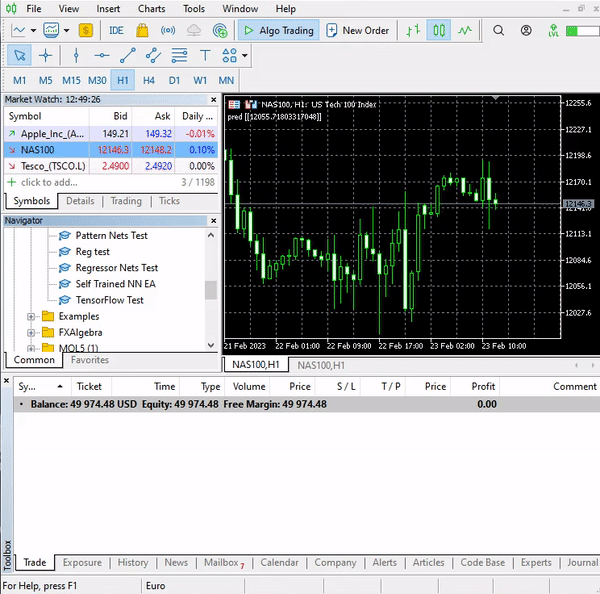### 策略测试器的结果

```CS      0       15:50:47.676    Tester  NAS100,H4 (Pepperstone-Demo): generating based on real ticks
CS      0       15:50:47.677    Tester  NAS100,H4: testing of Experts\Advisors\Self Trained NN EA.ex5 from 2023.01.01 00:00 to 2023.02.23 00:00 started with inputs:
CS      0       15:50:47.677    Tester    symbol_x=Apple_Inc_(AAPL.O)
CS      0       15:50:47.677    Tester    symbol_x2=Tesco_(TSCO.L)
CS      0       15:50:47.677    Tester    copy_rates_x=1
CS      0       15:50:47.677    Tester    n_samples=200
CS      0       15:50:47.677    Tester    =
CS      0       15:50:47.677    Tester    slippage=100
CS      0       15:50:47.677    Tester    stop_loss=2.0
CS      0       15:50:47.677    Tester    take_profit=2.0
CS      3       15:50:49.209    Ticks   NAS100 : 2023.02.21 23:59 - real ticks absent for 2 minutes out of 1379 total minute bars within a day

CS      0       15:50:51.466    History Tesco_(TSCO.L),H4: history begins from 2022.01.04 08:00
CS      0       15:50:51.467    Self Trained NN EA (NAS100,H4)  2023.01.03 01:00:00   [ 1/1000 ] Loss = 0.14025037 | accuracy -1.524
CS      0       15:50:51.468    Self Trained NN EA (NAS100,H4)  2023.01.03 01:00:00   [ 2/1000 ] Loss = 0.05244676 | accuracy 0.056
CS      0       15:50:51.468    Self Trained NN EA (NAS100,H4)  2023.01.03 01:00:00   [ 3/1000 ] Loss = 0.04488896 | accuracy 0.192
CS      0       15:50:51.468    Self Trained NN EA (NAS100,H4)  2023.01.03 01:00:00   [ 4/1000 ] Loss = 0.04114715 | accuracy 0.259
CS      0       15:50:51.468    Self Trained NN EA (NAS100,H4)  2023.01.03 01:00:00   [ 5/1000 ] Loss = 0.03877407 | accuracy 0.302
CS      0       15:50:51.469    Self Trained NN EA (NAS100,H4)  2023.01.03 01:00:00   [ 6/1000 ] Loss = 0.03725228 | accuracy 0.329
CS      0       15:50:51.469    Self Trained NN EA (NAS100,H4)  2023.01.03 01:00:00   [ 7/1000 ] Loss = 0.03627591 | accuracy 0.347
CS      0       15:50:51.469    Self Trained NN EA (NAS100,H4)  2023.01.03 01:00:00   [ 8/1000 ] Loss = 0.03564933 | accuracy 0.358
CS      0       15:50:51.470    Self Trained NN EA (NAS100,H4)  2023.01.03 01:00:00   [ 9/1000 ] Loss = 0.03524708 | accuracy 0.366
CS      0       15:50:51.470    Self Trained NN EA (NAS100,H4)  2023.01.03 01:00:00   [ 10/1000 ] Loss = 0.03498872 | accuracy 0.370

CS      0       15:50:51.662    Self Trained NN EA (NAS100,H4)  2023.01.03 01:00:00   [ 1000/1000 ] Loss = 0.03452066 | accuracy 0.379
CS      0       15:50:51.662    Self Trained NN EA (NAS100,H4)  2023.01.03 01:00:00   Testing Accuracy =0.717```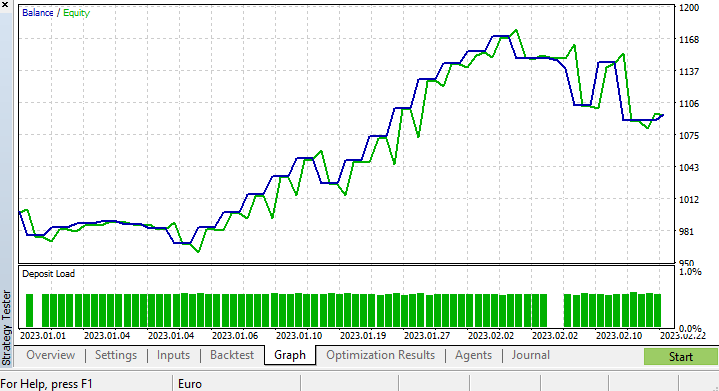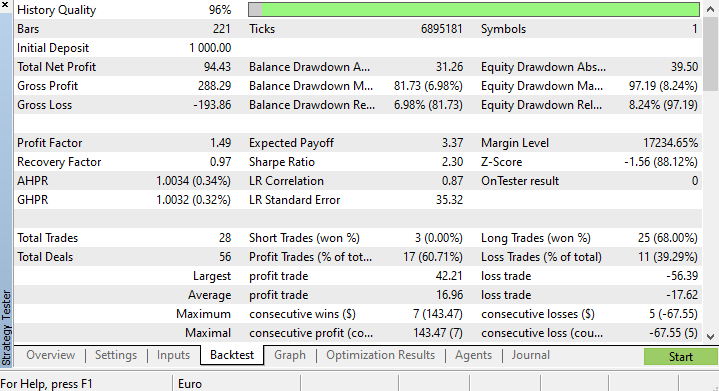```CS      0       17:11:52.100    Self Trained NN EA (NAS100,H4)  2023.01.03 01:00:00   [ 1000/1000 ] Loss = 0.05890808 | accuracy 0.615
CS      0       17:11:52.101    Self Trained NN EA (NAS100,H4)  2023.01.03 01:00:00   Testing Accuracy =0.635```

### 最后的思考metrics.mqh  包含测量神经网络模型准确性的函数。
preprocessing.mqh  包含缩放和准备神经网络模型数据的函数
matrix_utils.mqh  矩阵操控的附加函数
selftrain NN.mqh  包含自训练神经网络的主包含文件
Self Train NN EA.mq5  测试自训练神经网络的 EA

Attachments.zip (13.98 KB)

#### 该作者的其他文章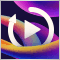开发回放系统 — 市场模拟（第 02 部分）：首次实验（II）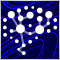神经网络实验（第 4 部分）：模板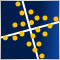数据科学和机器学习（第 13 部分）：配合主成分分析（PCA）改善您的金融市场分析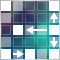神经网络变得轻松（第三十六部分）：关系强化学习